# Python | Get a list as input from user

We often encounter a situation when we need to take number/string as input from the user. In this article, we will see how to get as input a list from the user.

Examples:

```Input : n = 4,  ele = 1 2 3 4
Output :  [1, 2, 3, 4]

Input : n = 6, ele = 3 4 1 7 9 6
Output : [3, 4, 1, 7, 9, 6]
```

Code #1: Basic example

 `# creating an empty list ` `lst ``=` `[] ` ` `  `# number of elemetns as input ` `n ``=` `int``(``input``(``"Enter number of elements : "``)) ` ` `  `# iterating till the range ` `for` `i ``in` `range``(``0``, n): ` `    ``ele ``=` `int``(``input``()) ` ` `  `    ``lst.append(ele) ``# adding the element ` `     `  `print``(lst) `

Output: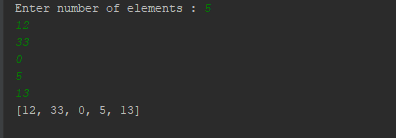Code #2: With handling exception

 `# try block to handle the exception ` `try``: ` `    ``my_list ``=` `[] ` `     `  `    ``while` `True``: ` `        ``my_list.append(``int``(``input``())) ` `         `  `# if the input is not-integer, just print the list ` `except``: ` `    ``print``(my_list) `

Output: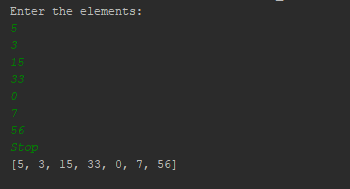Code #3: Using `map()`

 `# number of elements ` `n ``=` `int``(``input``(``"Enter number of elements : "``)) ` ` `  `# Below line read inputs from user using map() function  ` `a ``=` `list``(``map``(``int``,``input``(``"\nEnter the numbers : "``).strip().split()))[:n] ` ` `  `print``(``"\nList is - "``, a) `

Output: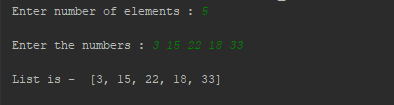Code #4: List of lists as input

 `lst ``=` `[ ] ` `n ``=` `int``(``input``(``"Enter number of elements : "``)) ` ` `  `for` `i ``in` `range``(``0``, n): ` `    ``ele ``=` `[``input``(), ``int``(``input``())] ` `    ``lst.append(ele) ` `     `  `print``(lst) `

Output: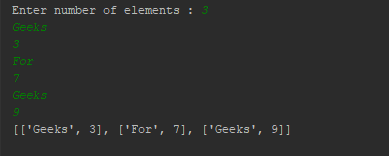Code #5: Using List Comprehension and Typecasting

 `# For list of integers ` `lst1 ``=` `[]   ` ` `  `# For list of strings/chars ` `lst2 ``=` `[]   ` ` `  `lst1 ``=` `[``int``(item) ``for` `item ``in` `input``(``"Enter the list items : "``).split()] ` ` `  `lst2 ``=` `[item ``for` `item ``in` `input``(``"Enter the list items : "``).split()] ` ` `  `print``(lst1) ` `print``(lst2) `

Output: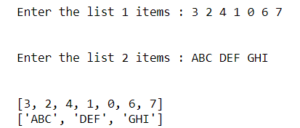Attention geek! Strengthen your foundations with the Python Programming Foundation Course and learn the basics.

To begin with, your interview preparations Enhance your Data Structures concepts with the Python DS Course.

My Personal Notes arrow_drop_upCheck out this Author's contributed articles.

If you like GeeksforGeeks and would like to contribute, you can also write an article using contribute.geeksforgeeks.org or mail your article to contribute@geeksforgeeks.org. See your article appearing on the GeeksforGeeks main page and help other Geeks.

Please Improve this article if you find anything incorrect by clicking on the "Improve Article" button below.

Improved By : rahulsrivastava7

Article Tags :
Practice Tags :

16

Please write to us at contribute@geeksforgeeks.org to report any issue with the above content.